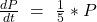## The population of a certain species of insect is given by a differentiable function P, where P(t) is the number of insects in the population

Question

The population of a certain species of insect is given by a differentiable function P, where P(t) is the number of insects in the population, in millions, at time t, where t is measured in days. When the environmental conditions are right, the population increases with resect to time at a rate that is directly proportional to the population. Starting August 15, the conditions were favorable and the. population began increasing. On August 20, five days later, there were an estimated 10 million insects and the population was increasing at a rate of 2 million insects per day.

Required:
What is the differential equation that models this situation?

in progress 0
4 months 2021-07-17T08:49:48+00:00 1 Answers 0 views 0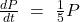Step-by-step explanation:

Given

Variation: Directly Proportional

Insects = 10 million when Rate = 2 million

i.e.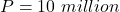when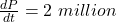Required

Determine the differential equation for the scenario

The variation can be represented as: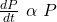Which means that the rate at which the insects increase with time is directly proportional to the number of insects

Convert to equation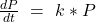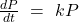Substitute values for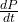and P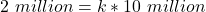Make k the subject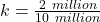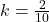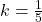Substitutefor k in